beginning of content:

People often think that to find solutions of differential equations, you simply find an antiderivative and then use an initial condition to evaluate the constant. While this gives a start to finding solutions of initial value problems, consideration must also be given to the domain of your final result. Sometimes these considerations are obvious, as in AB6 from the 2000 AP Exam, whose solution is given below.

## Example 1

The solution of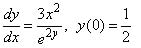is obtained by separating variables and finding an antiderivative as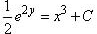, or, as this requires that x3 + C must always be positive,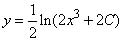.

Choosing C = e/2 allows the initial condition to be satisfied, and we have the solution of this initial value problem. The requirement that 2x3 + e > 0, or equivalently x > -(e/2)^(1/3), is a natural condition to have the logarithm function defined, so it includes the initial value and avoids the singularity.

However, finding solutions of initial value problems for separable differential equations need not always be as straightforward, as we see in our following four examples.

## Example 2

As a first such example, consider the initial value problem: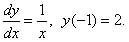All antiderivatives may be written as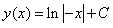,

(1)

and if C = 2, the initial condition is satisfied. However, even though this function satisfies the differential equation and initial value problem, it is NOT the solution of this differential equation. The solution is: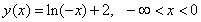.

(2)

The reason for this is that the usual definition of a solution of a differential equation is that of a differentiable function on an open interval that contains the initial x-value. Notice that the function in (1) is also defined for x > 0, while our solution must be continuous on an open interval containing the initial value at x = -1. Thus the domain of our solution cannot contain x = 0, or positive values, but must include x = -1. This requires the domain to be the open interval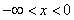.

## Example 3

As a second example where we need to be careful, consider the initial value problem: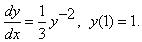Separating variables gives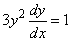, and finding antiderivatives gives y3 = x + C.

(3)

ChoosingC = 0 satisfies the initial condition. We usually like to find explicit solutions, so solving for y(x) gives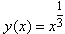.

(4)

We notice that this function does not have a derivative at x = 0, so we must add the condition x > 0 to our equation in (4) in order for it include x = 1 and be a solution of our original initial value problem. Thus the domain for our solution in (4) is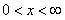. If our initial condition was y(-1) = -1, our solution would be (4) with the domain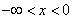.

## Example 4

For another example where we need to limit the domain of our solution, consider: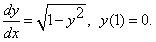If we separate variables and find antiderivatives, we obtain arcsin (y) = x + C.

(5)

Choosing C = -1 allows the initial condition to be satisfied. If we find an explicit solution by solving for y, we find y(x) = sin(x- 1),

(6)

and this presents a problem. From our original differential equation, and the slope field in Figure 1 below, we see that our solution should never have a negative value for its derivative, whereas our solution in (6) is oscillatory. The problem occurred when we solved equation (5) for y(x). In (6) we need to limit the domain of our solution to an interval of length π that includes our initial value and on which the initial sine graph is increasing.

Thus the correct solution to our problem is: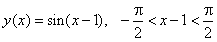.

Notice that this domain may also be written as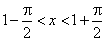.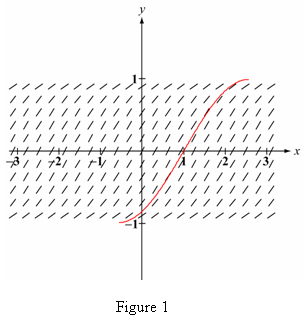## Example 5

As a final example to illustrate the need to limit the domain of an antiderivative in order to have it be a solution of a differential equation, consider: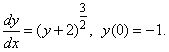Separating variables and finding antiderivatives gives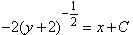,

(7)

so the initial condition is satisfied if C = -2. Solving (7) for y(x) gives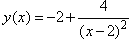.

(8)

We notice that (8) is valid for all x not equal to 2, and that this solution will have negative slopes for x > 2, while our original differential equation requires non-negative slopes everywhere. (A look at the slope field in Figure 2 below also demonstrates this fact.)

We got into this trouble when we squared both sides of (7) to obtain our explicit solution. Because the left side of (7) is always negative, we must limit the right side to x + C < 0. Thus we need to add the condition x < 2 to our solution in (8).

Notice that we could not consider x > 2 in any case, since the domain must be an open interval containing our initial value x = 0, and not include x = 2, where the solution is not defined. This example shows that we may not extend a solution across a discontinuity, even if the resulting function formally satisfies the differential equation on the other side of the discontinuity.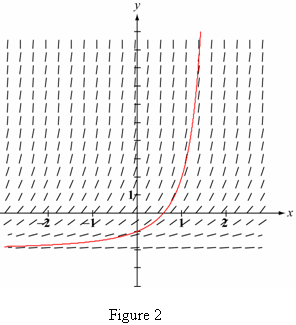## Conclusion

So what can we conclude from these examples?

1. The process of obtaining an explicit solution from an implicit solution may result in an incorrect solution of our initial value problem.
2. There may be values of x where the derivative of the explicit solution does not exist, even though it formally satisfies the differential equation.

To avoid these mistakes, we can always check to make sure that the explicit solution is such that:

• For all parts of the domain, the derivative of the explicit solution does not contradict the original differential equation. (Comparing the slope field for the differential equation with the graph of the explicit solution will display any differences.)
• Its derivative exists for all values in its domain.

For more information, see sections 1.1 and 2.4 of Lomen and Lovelock, Differential Equations: Data, Models, and Graphics, John Wiley and Sons, 1999.

The contributions of Larry Riddle, Ben Klein, and David Bressoud to this article were greatly appreciated.

David Lomen has taught mathematics for 45 years, and is currently a University Distinguished Professor at the University of Arizona. He has served as an AP Reader and workshop consultant for many years, and is currently a member of the AP Calculus Development Committee. He has authored or co-authored 39 research articles on applied mathematics, 17 educational articles, and 6 textbooks (on algebra, calculus, differential equations, and linear algebra). He has also given over three dozen workshops on teaching calculus and teaching with technology, and was the chairman of the National Advisory Committee for Systemic Teaching Excellence Projects to the state of Montana and the commonwealth of Puerto Rico.

## Authored by

• David Lomen
University of Arizona
Tucson, Arizona